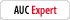# calculating age

The X
Registered: Mar 27 2011
Posts: 24

I have these two text fields representing dates. The first one is today's date and the second one is the user's birth date. They must input data in yyyymmdd format but I do not have any special script for my form to understand that data as dates. It's just a string of numbers.

Now what I need is to calculate the age of the user by substracting these two dates.

Based on that result, I want either one of two checkboxes to get checked if the user is minor.

My Product Information:
Acrobat Pro 10.0, Windows
thompRegistered: Feb 15 2006
Posts: 4411
Search on this site for "calculate age", using the search box above. You'll find loads.

Thom Parker
The source for PDF Scripting Info
www.pdfscripting.com
Very Important - How to Debug Your Script

gkaiserilRegistered: Feb 23 2006
Posts: 4307
You might want to consider using fields with date formats so you do not need to perform the validation of the imputed dates.

George Kaiser

The X
Registered: Mar 27 2011
Posts: 24
gkaiseril wrote:
You might want to consider using fields with date formats so you do not need to perform the validation of the imputed dates.
Ok so I did just that using yyyymmdd for both and tried this script without success:
// define some time constants
var fSec = 1000;//second
var fMin = 60 * fSec;// minute
var fHr = 60 * fMin;// hour
var fDay = 24 * fHr;// day
var fYear = 365.25 * fDay; // year

var strStart = this.getField("dob").value;
var strToday = this.getField("date").value;

// compute if there are values for dob and date
if(strStart.length && strToday.length) {var dob = util.scand("yyyymmdd", strStart);// convert dob to date time object
var date = util.scand("yyyymmdd", strToday);// convert date to date time object
var diff = date.valueOf() - dob.valueOf();// compute difference of values
event.value = Math.floor(diff/ fYear);// truncate to whole years}

I assume this calculates what I want but how do I put the result in a variable so I can put it in "age" text field?

thompRegistered: Feb 15 2006
Posts: 4411
The script you've posted is for a calculation event on a text field in an AcroForm, i.e., it should be placed in the calculate script for the field where the age will be displayed.

To set a check box field add this code to the end:

var bOverAge = (event.value > 18);
this.getField("MyFirstCheck").checkThisBox(0,bOverAge);
this.getField("MySecondCheck").checkThisBox(0,!bOverAge);

the code is slightly different if you mean radio buttons instead of check boxes.

Thom Parker
The source for PDF Scripting Info
www.pdfscripting.com
Very Important - How to Debug Your Script

gkaiserilRegistered: Feb 23 2006
Posts: 4307
By using the custom format for the date and not including any non-numeric separators you have instructed JavaScript to treat the value of the fields as numeric values and not string values. If you check the length of numeric value, you will always get a value of zero. You need to convert any numeric value to a string in order to obtain the length property of the string of numbers that represent your numeric value. Also if you remove the 'if' statement, the util.scand() method will not work because you are supplying a numeric value for the date string and not a character string value.

The following code adjust for those issues and includes today in the age calculation.

// define some time constants
var fSec = 1000;//second
var fMin = 60 * fSec;// minute
var fHr = 60 * fMin;// hour
var fDay = 24 * fHr;// day
var fYear = 365.25 * fDay; // year

var strStart = this.getField("dob").value;
var strToday = this.getField("date").value;

var AgeYears = ""; // clear field in case there is not calculation
// compute if there are values for dob and date
if(strStart != "" & strToday != "") {
var dob = util.scand("yyyymmdd", strStart.toString());// convert dob to date time object
var date = util.scand("yyyymmdd", String(strToday));// convert date to date time object
var diff = date.valueOf() - dob.valueOf();// compute difference of values
diff = diff + fDay // adjust to include today in age calculation
AgeYears = Math.floor(diff / fYear);// truncate to whole years
}
// set field value or other action based on value of AgeYears
event.value = AgeYears; // or you can specify another field value to set

George Kaiser

The X
Registered: Mar 27 2011
Posts: 24
Great, it's almost it.

gkaiseril: the calculation is exact and it displays the right age in the text box but I don't want that "age" text box. I just used it to test the calculation. I want the calculation to perform itself and give the answer via check boxes. Since there is no calculate script in check boxes, what do I do? I kept the "age" checkbox but set it as hidden from the user. tadaamm.

thomp: I added "=" to ">" because otherwise, it would not take 18 as an adult. It works fantastic. thanx a lot.
gkaiserilRegistered: Feb 23 2006
Posts: 4307
Minor is a legal term and can very from country to country and state to state. You will need to provide more information. You may also need to provide for an emancipated minor.

You can use a 'if' statement of 'switch' statement to control the flow of the JavaScript calculation.

George Kaiser